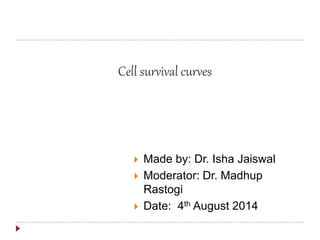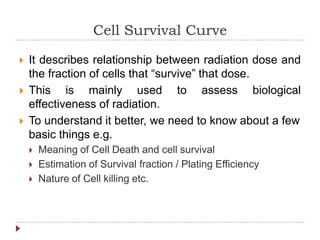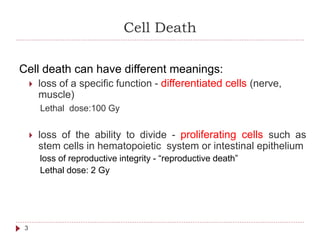Seu SlideShare está sendo baixado. ×

# Cell survival curve

Anúncio
Anúncio
Anúncio
Anúncio
Anúncio
Anúncio
Anúncio
Anúncio
Anúncio
Anúncio
AnúncioCarregando em…3
×

1 de 49 Anúncio

# Cell survival curve

describes relationship between radiation dose and the fraction of cells that “survive” that dose

model of cell killing
target model

describes relationship between radiation dose and the fraction of cells that “survive” that dose

model of cell killing
target model

Anúncio
Anúncio

### Cell survival curve

1. 1.  Made by: Dr. Isha Jaiswal  Moderator: Dr. Madhup Rastogi  Date: 4th August 2014 Cell survival curves
2. 2. Cell Survival Curve  It describes relationship between radiation dose and the fraction of cells that “survive” that dose.  This is mainly used to assess biological effectiveness of radiation.  To understand it better, we need to know about a few basic things e.g.  Meaning of Cell Death and cell survival  Estimation of Survival fraction / Plating Efficiency  Nature of Cell killing etc.
3. 3. Cell Death 3 Cell death can have different meanings:  loss of a specific function - differentiated cells (nerve, muscle) Lethal dose:100 Gy  loss of the ability to divide - proliferating cells such as stem cells in hematopoietic system or intestinal epithelium loss of reproductive integrity - “reproductive death” Lethal dose: 2 Gy
4. 4. Survival 4  “Survival” means retention of reproductive integrity  ie. the capacity for sustained proliferation  Can grow into macroscopic colonies  A SURVIVOR that has retained its reproductive integrity and is able to proliferate indefinitely is said to be CLONOGENIC
5. 5. Estimating Survival 5  In order to determine the surviving fraction, we must know the PLATING EFFICIENCY  PE is the percentage of cells that grow into colonies  in other words, those cells that survive the plating process  may be close to 100% in some established cell lines but 1% or less for fresh explants of human cell
6. 6. Derivation of Survival Curves 6  Cells have been taken from stock culture and placed in seed dishes  Then irradiated (0 Gy to 6 Gy)and allowed to grow into colonies for 1-2 weeks  Colonies have been counted for survival data Always will have a control batch to determine PE.
7. 7. Surviving Fraction  Equal to the fraction of cells that plate successfully and survive irradiation (without losing their reproductive integrity) to grow into colonies  A cell survival curve is graph plotted between surviving fraction on Y axis & absorbed dose on X axis  PE/100seededcells countedColonies fractionSurviving   7
8. 8. 8 Dose (Gy) Surviving Fraction 2 64 0.01 0.1 1 0.001 SF = = 0.2 72 colonies 400 seeded x 0.9 plated
9. 9. Modes of Radiation Injury • Primarily by ionization (direct) and free radicals(indirect) mechanism • Low LET (X- and gamma-rays) damage by free radicals • High LET (protons and a particles) damage by ionization
10. 10.  The Poisson model was proposed in 1961 (Munro & Gilbert)  It states“the object of treating a tumour by radiotherapy is to damage every single potentially malignant cell to such an extent that it cannot continue to proliferate.”  Poisson statistics are used to determine the probability of an event occurring in relation to the known average number of events occurring.(λ)  When enough radiation is delivered to the tumour mass such that 1 lethal hit would be expected per cell,…  the likely percentage of cells receiving one lethal hits is 63%. Therefore, 37% of cells would receive no lethal hits and therefore the tumour would most likely not be cured.  The probability of zero surviving tumor cell is given by the tumour control probability (TCP)  Poisson Model of probability of cell death
11. 11.  As no of lethal hits increases the probability of survival decreasing geometrically with dose.  For example, if 100 clonagens were present within the tumour, an average of 37 clonagens would survive. Therefore the TCP would be e-37  As the lethal hits per clonagen increases, the TCP also increases.  The increase occurs rapidly once the lethal hits per clonagen exceeds 3 – 4 (likelihood of surviving clonagens begins to approach zero at this point).
12. 12. Quantization of cell killing  A dose of radiation that introduces an average of one lethal event per cell leaves 37% still viable is called D0 dose.  Cell killing follows exponential relationship. A dose which reduces cell survival to 50% will, if repeated, reduce survival to 25%, and similarly to 12.5% from a third exposure.  This means Surviving fraction never becomes zero.
13. 13. Log-linear scale: straight line in invitro cell culture  A straight line results when cell survival (from a series of equal dose fractions) is plotted on a logarithmic scale as a function of dose on linear scale.  The slope of such a semi- logarithmic dose curve could be described by the D0, the dose to reduce survival to 37%,  D0 usually lies between 1 and 2 Gy  D50, the dose to reduce survival to 50%,  D10, the dose to reduce survival to 10%. D10= 2.3 x D0
14. 14. Mammalian cell Survival Curve Shape  Two Component: shoulder and exponential curve  Initial portion has a shoulder and terminal portion become straight line.  In low dose region ,some dose of radiation goes waste to overcome some threshold  Terminal portion follow exponential relationship means same dose increment result into equal reduction in surviving fraction.
15. 15. Mammalian Cell Survival Curve  Shoulder Region  Shows accumulation of SUB- LETHAL DAMAGE.  The larger the shoulder region, the more dose will initially be needed to kill the same proportion of cells, so less radiosensitive  Beyond shoulder region  The D0 dose, or the inverse of the slop of the curve, indicates the relative radio sensitivity. The smaller the D0 dose, the greater the radio sensitivity.
16. 16. Models of Description of the Curve  Target theory: Single-target Model Multi-target Model  Linear Quadratics Model
17. 17. Single Target Single Hit Inactivation Model  single hit on a single target within the cell leads to cell death.  This generates an exponential cell survival curve which appears as a straight line on a semi- logarithmic scale.  This model is useful for highly sensitive human tissues, if high LET radiation is used,  Mammalian cells usually have a shoulder on their cell survival curves, which is not seen in this model. If, at dose D0 there is an average of one lethal event/cell, then S = e-D/D0 where D0 is called the mean lethal dose Simple Target Theory: ln S = -D/D0
18. 18. Multi-target Model  in an attempt to explain the shoulder of the cell survival curve, this model was generated.  It proposes that a single hit to each of n sensitive targets within the cell is sufficient to cause cell death.  The generated curve has a shoulder and decreases linearly with increasing dose.
19. 19. Multi-Target Model 20 Dose, Gy 100 10-1 10-4 0 123 6 9 Survival 10-3 10-2 Initial slope measure, D1, due to single-event killing Final slope measure, D0, due to multiple-event killing Dq n n or Dq represents the size or width of the shoulder Quantified in terms of  measure of initial slope due to single- event killing, D1  measure of final slope due to multiple-event killing, D0  width of the shoulder, Dq or n
20. 20. Multi-target Model 21  Shoulder-width measures:  the quasi-threshold dose (Dq)  the dose at which the extrapolated line from the straight portion of the survival curve (final slope) crosses the axis at 100% survival  the extrapolation number (n)  This value is obtained by extrapolating the exponential portion of the curve to the vertical line.  “broad shoulder” results in larger value of n  “narrow shoulder” results in small value of n  n = exp[Dq / D0]
21. 21. Single-target, single hit/multi-target, single hit model  Major problem with this model is that there are too many parameters D1;D0;Dq  Need a mathematically simpler model with fewer “unknown” parameters  The linear-quadratic (L-Q) model meets these needs
22. 22. Linear-quadratic model The linear quadratic model uses a polynomial equation (αD+βD2). The probability of survival is equal to the exponential of this – ie:S = e−(αD+βD)2. The generated curve is perhaps the best approximation of the actual cell kill seen after radiation exposure. It has the added benefit of two constants (α and β) which can be determined for specific tissues and cancers to predict dose response
23. 23. L-Q Model
24. 24. Linear Quadratic Model 25  S = e-(aD + bD 2 )  where:  S represents the fraction of cells surviving  D represents dose  a and b are constants that characterize the slopes of the two l portions of the semi-log survival curve  biological endpoint is cell death
25. 25. Linear Quadratic Model 26  Linear and quadratic contributions to cell killing are equal when the dose is equal to the ratio of a to b  D = a/b or  aD = b D2  a component is representative of damage caused by a single event (hit, double-strand break, “initiation / promotion” etc.)  b component is representative of damage caused by multiple events (hit/hit, 2 strand breaks, initiation then promotion, etc.)
26. 26. Comparison of the L-Q and target theory models
27. 27. Comparison of the L-Q and target theory models  Neither the L-Q not the M-T model has any established biological basis.  L-Q curves retain curvature even at very high doses  Target Theory curves become linear at high doses  With high-LET radiations, both theories result in straight lines ( irreparable damage dominates)
28. 28. Linear –quadratic model Multi-target model  At high doses the LQ model predicts a survival curve that bends continuosly, whereas the M-T model become linear.  At low doses the LQ model describes a curve that bends more than a M-T curve.
29. 29. Factors affecting cell survival curve 1. Mechanism of cell death 2. LET 3. Fractionation 4. Dose rate effect 5. Intrinsic radio sesitivity 6. Cell cycle stage 7. Oxygen presence
30. 30. Mitotic death results (principally) from exchange-type chromosomal aberration.  survival is linear quadratic function of dose Survival curve is og-linear plot with broad shoulder  Characterized by dose-rate effect Apoptotic death result from programmed death.  straight line on log-linear plot.  Characterized by exponential function of dose. Survival curve is straigh & shoulderless  little or no dose-rate effect. 1:SURVIVAL-CURVE SHAPE AND MECHANISM OF CELL DEATH
31. 31. Survival-curve shape and mechanism of cell death mitotic death Most cells fall between apopototic & mitotic death Note! Shoulder
32. 32. 2:LINEAR ENERGY TRANSFER 33  Rate at which energy is transferred to cell during irradiation  Low-LET radiations:  At low dose region  shoulder region appears  At high dose region  survival curve becomes linear and surviving fraction to an exponential function of dose  surviving fraction is a dual exponential S = e-(aD+bD2)
33. 33. 34  High-LET radiations:  survival curve is linear  surviving fraction is a pure exponential function of dose S = e-(aD)
34. 34. Survival Curves and LET 35  Increasing LET:  increases the steepness of the survival curve  results in a more linear curve  shoulder disappears due to increase of killing by single- events
35. 35. 3:Fractionation  If the dose is delivered as equal fractions with sufficient time ,repair of sub-lethal damage ocurs  Elkind’ s Recovery takes place between radiation exposure , cell act as fresh target.  Elkind & Sutton showed that when two exposure were given few hours apart ,the shoulder reappeared. Dq Dq Dose (Gy) 5 10 15 20 25 104 103 102 101 100 10-1 10-2 D0 n = exp[Dq / D0]
36. 36. The Effective Survival Curve: Fractionation 37  If the dose is delivered as equal fractions with sufficient time between for repair of the sub-lethal (non-killing) damage, the shoulder of the survival curve is repeated many times.  The effective survival curve becomes a composite of all the shoulder repetitions.  Dose required to produce the same reduction in surviving fraction increases. Showing ~28 Gy in 14 fractions.
37. 37. 4 :Dose-rate effect Dose rate determines biological impact At low dose rates, DNA repair processes are able to repair sub lethal damage during the radiation treatment. At very low dose rates other radiobiological effects (re-oxygenation, redistribution and repopulation) may also begin to play a role. This lessens the effect of a particular dose of radiation. Low dose rates tend to negate the β component of the linear quadratic equation, and the line becomes straighter on a logarithmic scale. reduction in dose rate generally reduces survival-curve slope (D0 increases) .
38. 38. Dose-Rate Effect in CHO Cells
39. 39. Composite survival curves for 40 Human Cell Lines Survival Curves show greater variation, Greater range of repair Less variation in cell survival curve due to less repair
40. 40. 5:Intrinsic radiosensitivity  Due to the differences in DNA content  represents bigger target for radiation damage  Sterilizing radiation dose for bacteria is 20,000 Gy wheras for mammalian cell is 1-2 Gy Mammalian cells are significantly more radio-sensitive than microorganisms:
41. 41. 6:survival curve: effect of cell cycle stage Late S: least senstive. M>G2>G1>early S>late S for sensitivity Difference caused by cell cycle are similar to difference caused by Oxygen effect The range of senstivity between the most senstive (m) & most resistant (s) phase is of the same order as oxygen effect The broken line is cell survival curve for mitotic cell plotted under hypoxia Slope is 2.5 times shallower than aerated cell
42. 42.  Cells are most sensitive to radiation at or close to M  Cells are most resistant to radiation in late S  For prolonged G1phase  a resistant period is evident in early G1 followed be a sensitive period in late G1  Cells are usually sensitive to radiation in G2 (almost as sensitive as in M)
43. 43.  Oxygen modifies the biological effects of ionizing radiation  OER – oxygen enhancement ratio: ratio of hypoxic doses : aerated doses needed to achieve the same biological effect  OER is absent for high LET radiations like alpha-particles and is intermediate for fast neutron.  Low LET radiation (eg. photons, electrons) are highly dependent on the presence of oxygen to ‘fix’ damage caused by radicalised DNA.  The oxygen effect shows more cell killing in oxic conditions. This is seen in cell survival curves as a shift in the steepness of the curve  For low LET X-Rays/γ-Rays  at high doses OER is 2.5-3.5 at lower doses OER is ~2.5 7: The Oxygen Effect
44. 44. OER – oxygen enhancement ratio: ratio of hypoxic doses : aerated doses needed to achieve the same biological effect
45. 45. Summary  A cell survival curve is the relationship between the fraction of cells retaining their reproductive integrity and absorbed dose.  Conventionally, surviving fraction on a logarithmic scale is plotted on the Y-axis, the dose is on the X-axis . The shape of the survival curve is important.  The cell-survival curve for densely ionizing radiations (α- particles and low-energy neutrons) is a straight line on a log- linear plot, that is survival is an exponential function of dose.  The cell-survival curve for sparsely ionizing radiations (X-rays, gamma-rays has an initial slope, followed by a shoulder after which it tends to straighten again at higher doses.
46. 46.  Survival data are fitted by many models. Some of them are: multitarget hypothesis, linear-quadratic hypothesis etc  At low doses and for single fraction regimen most cell killing results from “α-type” (single-hit, non-repairable) injury, but that as the dose increases, the“β –type” (multi-hit, repairable) injury becomes predominant, increasing as the square of the dose.  Initial slope of cell survival curve determined by α.  causes the curve to bend at higher doses.  The survival curve for a multifraction regimen is also an exponential function of dose.  The D10, the dose resulting in one decade of cell killing, is related to the Do by the expression D10 = 2.3 x Do Summary
47. 47. Cell survival curve depends Factors that make cells less radiosensitive are: Cell survival curve is used to calculate 1. on nature of radiation (LET); 2. Type of cell death 3. dose; 4. dose rate 5. cell type; 6. cell cycle stage 7. oxygen presence 1. removal of oxygen to create a hypoxic state, 2. the addition of chemical radical scavengers, 3. the use of low dose rates or multif ractionated irradiation, 4. cells synchronized in the late S phase of the cell cycle. 1. no.of tumor cell killed/ survived 2. tumor control probability 3. calculation of time dose and fractions 4. calculating Biologically effective dose
48. 48. Thank You